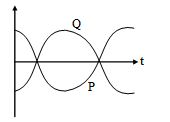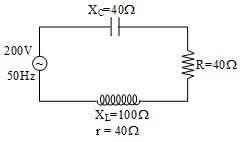﻿ AC - Alternating Current Set 2, NCERT Physics Class 12 - MCQ Quiz by Dr. Mukesh Shrimali

# Topic: AC - Alternating Current Set 2, Physics MCQ Quiz Test

#### How to Count Your Score

You will get 1 point for each correct answer. At the end of the Quiz, your total score will be displayed. Maximum score is 50 points.

Good Luck, Lets start the Quiz on Physics Topic AC - Alternating Current Set 2

MCQ 1:
An alternating current changes from a complete cycle in 1μs, then the frequency in Hz will be -

MCQ 2:
An alternating voltage source is connected, in an A.C. circuit whose maximum value is 170 volt. The value of potential at a phase angle of 45° will be -

MCQ 3:
In an ac circuit, the current is given by i = 4 sin (100πt + 30°) ampere. The current becomes maximum first time (after t = 0) at t equal to -

MCQ 4:
The instantaneous value of current in an ac circuit is I = 2 sin (100πt + π/3) A. The current at the beginning (t = 0) will be -

MCQ 5:
In A.C. circuit the average value per cycle of e.m.f. or current is -

MCQ 6:
Ratio of rms & average value of alternating signal (form factor) -

MCQ 7:
The r.m.s. value of potential due to superposition of given two alternating potentials E1 = E0 sin ωt and E2 = E0 cos ωt will be -

MCQ 8:
If the value of Erms is 5 volt, then the amplitude of emf in volt is -

MCQ 9:
A mixer of 1000Ω resistance is connected to an A.C. source of 200V and 50 cycle/sec. Then the value of average potential difference across the mixer will be -

MCQ 10:
If instantaneous value of current is I = 10 sin (314 t) A, then the average current for the half cycle will be -

MCQ 11:
The r.m.s. value of alternating current is 10 amp having frequency of 50 Hz. The time taken by the current to increase from zero to maximum and the maximum value of current will be -

MCQ 12:
In a circuit an a.c. current and a d. c. current are supplied together. The expression of the instantaneous current is given as i = 3 + 6 sin ωt. Then the rms value of the current is -

MCQ 13:
The emf and the current in a circuit are E = 12 sin (100πt) and I = 4 sin (100πt + π/ 3) then -

MCQ 14:
The direction of alternating current get changed in one cycle

MCQ 15:
If the frequency of alternating potential is 50Hz then the direction of potential, changes in one second by -

MCQ 16:
The time period of alternating current with frequency of one KHz one second will be -

MCQ 18:
The value of alternating e.m.f. is e = 500 sin 100πt , then the frequency of this potential in Hz is -

MCQ 18:
The frequency of an alternating current is 50Hz, then the time to complete one cycle for current vector will be-

MCQ 19:
In the above question, time taken by current to rise from zero to maximum is -

MCQ 20:
In the equation for A.C. I = I0 sin ωt, the current amplitude and frequency will respectively be -

MCQ 21:
The sinusoidal voltage wave changes from 0 to maximum value of 100 volt. The voltage when the phase angle is 30° will be -

MCQ 22:
If the frequency of ac is 60 Hz the time difference corresponding to a phase difference of 60° is -

MCQ 23:
The domestic power supply is at 220 volt. The amplitude of emf will be -

MCQ 24:
The phase difference between the current and the electromotive force in an ac circuit is ?/4 radian. If the frequency is 50 Hz, then the time difference corresponding to this phase difference, will be -

MCQ 25:
In A.C. circuit the ratio of virtual current and the r.m.s. current is –

MCQ 26:
If the r.m.s. value of A.C. is Irms then peak to peak value is -

MCQ 27:
The average value or alternating current for half cycle in terms of I0 is -

MCQ 28:
Sinusoidal peak potential is 200 volt with frequency 50Hz. It is represented by the equation -

MCQ 29:
If the instantaneous value of currents is I = 100 sin 314t Amp. then the average of current in Ampere for half cycle is -

MCQ 30:
The equation of current in an ac circuit is I = 4 sin (100πt + π/6) ampere. The current at the beginning (t = 0) will be -

MCQ 31:
RMS value of ac i = i1 cos ωt + i2 sin ωt will be -

MCQ 32:
The phase difference between the alternating current and voltage represented by the following equation I = I0 sin ωt, E = E0 cos (ω t + π/3), will be -

MCQ 33:
The inductance of a resistance less coil is 0.5 Henry. In the coil the value of A.C. is 0.2 Amp whose frequency is 50Hz. The reactance of circuit is -

MCQ 34:
The inductive reactance of a coil is 1000Ω. If its self inductance and frequency both are increased two times then inductive reactance will be -

MCQ 35:
In an L-C-R series circuit R = 10 Ω, XL = 8 Ω and XC = 6 Ω the total impedance of the circuit is -

MCQ 36:
In the given figure, the potential difference is shown on R, L and C. The e.m.f. of source in volt is -MCQ 37:
In an L.C.R series circuit R = 1 Ω, XL = 1000 Ω and XC = 1000 Ω. A source of 100 m.volt is connected in the circuit the current in the circuit is -

MCQ 38:
Which of the following figure showing the phase relationship is correct phase diagram for an R–C circuit-

MCQ 39:
A coil of inductance 0.1 H is connected to an alternating voltage generator of voltage E = 100 sin (100t) volt. The current flowing through the coil will be –

MCQ 40:
The vector diagram of the current and voltage in a given circuit is shown in the figure. The components of the circuit will be -MCQ 41:
Figure shows the variation of voltage with time for an ac I = I 0 sin ω t flowing through a circuit -MCQ 42:
The power factor of the following circuit will be -MCQ 43:
In a circuit, the reactance of a coil is 20Ω. If the inductance of the coil is 50 mH then angular frequency of the current will be -

MCQ 44:
If a capacitor is connected to two different A.C. generators then the value of capacitive reactance is -

MCQ 45:
Alternating current lead the applied e.m.f. by π/2 when the circuit consists of –

MCQ 46:
The reactance of a capacitor is X1 for frequency n1 and X2 for frequency n2 then X1 : X2 is -

MCQ 47:
A coil has reactance of 100Ω when frequency is 50Hz. If the frequency becomes 150Hz, then the reactance will be -

MCQ 48:
In pure inductive circuit, the curves between frequency f and inductive reactance 1/XL is -

MCQ 49:
In pure capacitive circuit if the frequency of A.C. is doubled, then the value of capacitive reactance will become -

MCQ 50:
In an A.C. circuit, a capacitor of 1μF value is connected to a source of frequency 1000 rad/sec. The value of capacitive reactance will be -